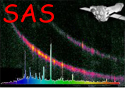XMM-Newton Science Analysis System

arfgen (arfgen-1.104) [xmmsas_20230412_1735-21.0.0]

## Encircled Energy Correction

• Rebin the energy grid used in the main stage by a factor eegridfactor

• For each energy

• Generate a model of the PSF centred at [xdet,ydet].

• Loop over each cell in a grid, with resolution given by badpixelresolution, calculating the PSF contribution in every cell. Add the cell contribution to a running total which finally gives the fraction of the PSF within the selected region for this energy

• Write encircled energy fraction as an element of an encircled energy fraction vector.

• Interpolate EE fraction vector to map to original energy grid used in main stage.

• Divide area vector by EE fraction vector.

Subsections

XMM-Newton SOC -- 2023-04-16